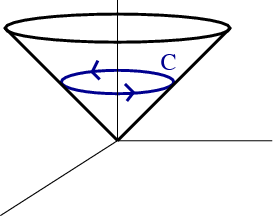# Math Insight

### Image: Curve formed from intersection of cone and a planeThe curve $\dlc$ formed from the intersection of the cone $z^2=x^2+y^2$ and the plane $z=1$.

Image file: curve_intersection_cone_plane.png